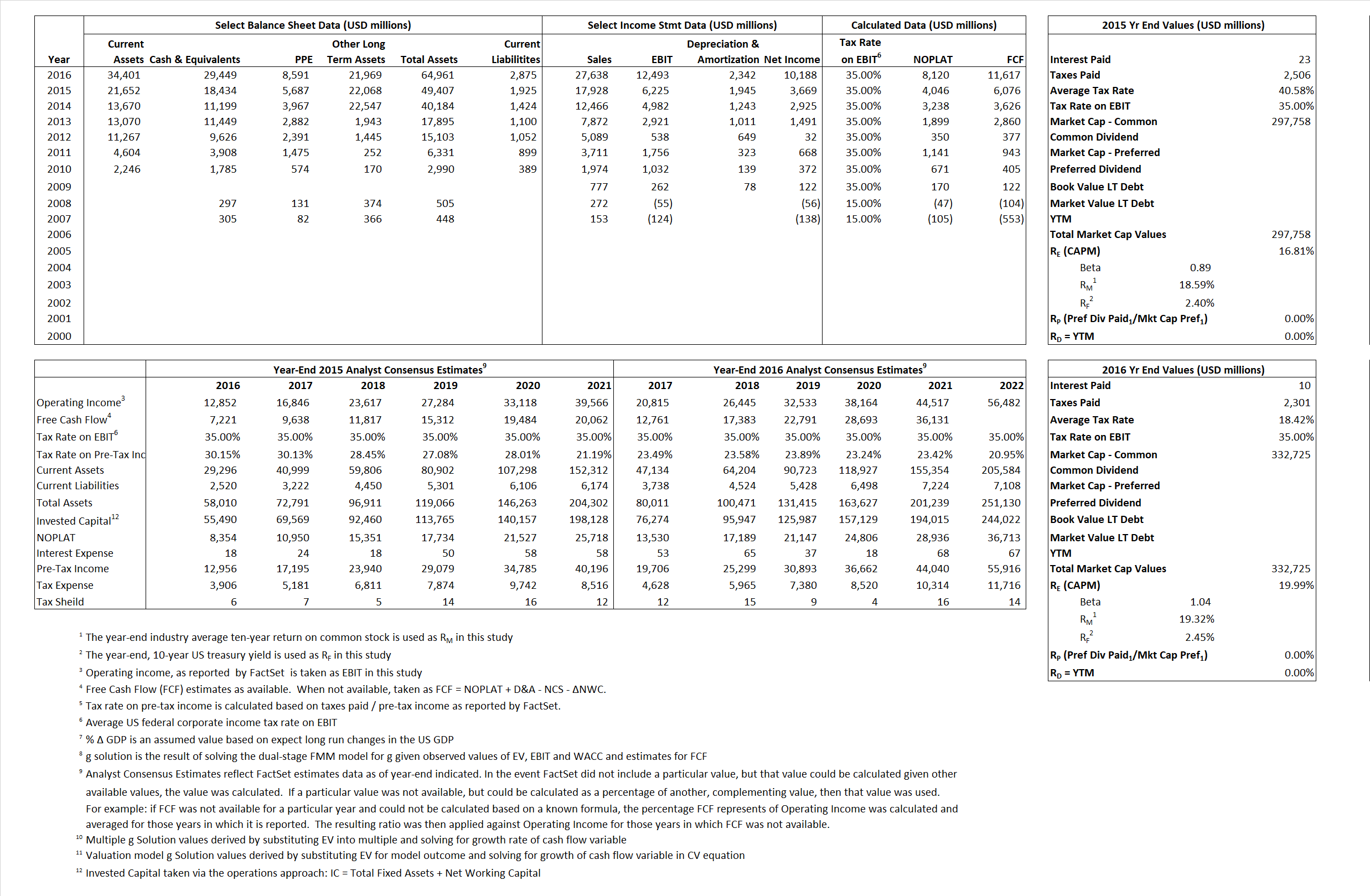## Analyst Listing

The following analysts provide coverage for the subject firm as of May 2016:

 Broker Analyst Analyst Email Raymond James Aaron Kessler aaron.kessler@raymondjames.com Nomura Research Anthony DiClemente anthony.diclemente@nomura.com CRT Capital Group Arvind Bhatia abhatia@sterneageecrt.com Sterne Agee & Leach Arvind Bhatia abhatia@sterneageecrt.com Pivotal Research Group Brian Wieser brian@pvtl.com Bernstein Research Carlos Kirjner carlos.kirjner@bernstein.com Atlantic Equities James Cordwell j.cordwell@atlantic-equities.com Oppenheimer Jason Helfstein jason.helfstein@opco.com Cowen & Company John Blackledge john.blackledge@cowen.com Needham Laura Martin lmartin@needhamco.com Rosenblatt Securities Martin Pyykkonen mpyykkonen@rblt.com Wedbush Securities Michael Pachter michael.pachter@wedbush.com Wells Fargo Securities Peter Stabler peter.stabler@wellsfargo.com William Blair Ralph Schackart rschackart@williamblair.com SunTrust Robinson Humphrey Robert S. Peck robert.peck@suntrust.com JMP Securities Ronald V. Josey rjosey@jmpsecurities.com Deutsche Bank Research Ross Sandler ross.sandler@db.com Stifel Nicolaus Scott W. Devitt swdevitt@stifel.com Susquehanna Financial Group Shyam Patil shyam.patil@sig.com Credit Suisse Stephen Ju stephen.ju@credit-suisse.com Hilliard Lyons Stephen Turner sturner@hilliard.com Cantor Fitzgerald Youssef H. Squali ysquali@cantor.com

## Primary Input Data## Derived Input Data

### Equational Form

Net Operating Profit Less Adjusted Taxes NOPLAT 4,046  8,120$NOPLAT\, =\, EBIT\, x\, (1 \,-\, Avg \,\,Tax\,\, Rate\,\, on\,\, EBIT)$
Free Cash Flow FCF 6,076 11,617$FCF\,=NOPLAT\,+\,Non-Cash\,Expenses-\Delta NWC\,-\,NCS$
Tax Shield TS 9 2$TS\,=\,Interest\,\,Paid\,\,x\,\, Avg \,\,Tax\,\,Rate\,\, on\,\, Pre-Tax\,\, Income$
Invested Capital IC 47,482 62,086$IC\,=\,Fixed\,\,Operating\,\,Assets\,\,+\,\,Net\,\, Working\,\, Capital$
Return on Invested Capital ROIC 8.52% 13.08%$ROIC\,=\,\frac { NOPLAT }{ IC }$
Net Investment NetInv 10,667 16,946$NetInv\,=\,{ {IC}_{1}}-{{IC}_{0}}+Depreciation$
Investment Rate IR 263.63% 208.68%$IR\,=\,\frac {NetInv}{NOPLAT}$
Weighted Average Cost of Capital
WACCMarket 16.81%  19.99%$WACC\,=\,\frac { E }{ V } { R }_{ E }\,+\,\frac { P }{ V } { R }_{ P }\,+\,\frac { D }{ V } { R }_{ D }\left( 1- Avg\,\, Tax\,\,Rate\,\,on\,\,Pre-Tax\,\,Income \right)$
WACCBook    8.58%    9.24%
Enterprise value
EVMarket 279,324 303,276$EV\,=\,Market\,\,Cap\,\,Equity\,+\,\,Long\,\,Term\,\,Debt\,-\,Cash$
EVBook  279,324  303,276
Long-Run Growth
g = IR x ROIC
22.47%   27.29% Long-run growth rates of the income variable are used in the Continuing Value portion of the valuation models.
g = %$\Delta$ GDP    2.50%    2.50%
Margin from Operations M   34.72%   45.20%$M\,\,=\,\,\frac{EBIT}{SALES}$
Depreciation/Amortization Rate D   23.81%   15.79%$D\,\,=\,\,\frac{D+A}{EBITDA}$

## Valuation Multiple Outcomes

The outcomes presented in this study are the result of original input data, derived data, and synthesized inputs.

### model g solution

12/31/2015 12/31/2016 12/31/2015 12/31/2016 12/31/2015 12/31/2016

EV/SALES$\frac {EV}{Sales} \,= \,\frac{ROIC\, -\, g}{ROIC\,(WACC\,-\,g)}\,(1\,-\,T)\,(M)$

15.58  10.97  18.51%  21.77%  17.63%  20.61%

EV/EBITDA$\frac {EV}{EBITDA} \,= \,\frac{ROIC\, -\, g}{ROIC\,(WACC\,-\,g)}\,(1\,-\,T)\,(1\,-\,D)$

34.19  20.44 18.51% 21.77% 17.63% 20.61%

EV/NOPLAT$\frac {EV}{NOPLAT} \,= \,\frac{ROIC\, -\, g}{ROIC\,(WACC\,-\,g)}$

69.03 37.35 18.51% 21.77% 17.63% 20.61%

EV/FCFOPS$\frac {EV}{FCF_{OPS}} \,= \,\frac{ROIC\, -\, g}{ROIC\,(WACC\,-\,g)}\,(1\,-\,T)$

45.97 26.11 18.51% 21.77% 17.63% 20.61%

EV/EBIT$\frac {EV}{EBIT} \,= \,\frac{ROIC\, -\, g}{ROIC\,(WACC\,-\,g)}\,(1\,-\,T)$

44.87 24.28 18.51% 21.77% 17.63% 20.61%

EV/IC$\frac {EV}{IC} \,= \,\frac{ROIC\, -\, g}{WACC\,-\,g}$

5.88  4.88 18.51% 21.77% 17.63% 20.61%